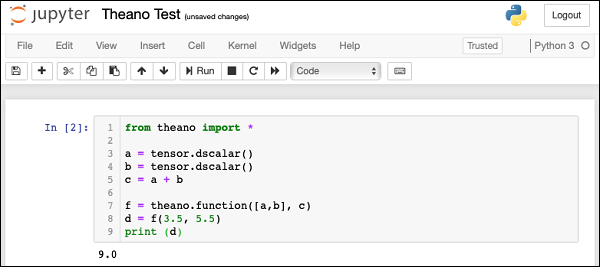# Theano - A Trivial Theano Expression

Let us begin our journey of Theano by defining and evaluating a trivial expression in Theano. Consider the following trivial expression that adds two scalars −

c = a + b

Where a, b are variables and c is the expression output. In Theano, defining and evaluating even this trivial expression is tricky.

Let us understand the steps to evaluate the above expression.

## Importing Theano

First, we need to import Theano library in our program, which we do using the following statement −

from theano import *


Rather than importing the individual packages, we have used * in the above statement to include all packages from the Theano library.

## Declaring Variables

Next, we will declare a variable called a using the following statement −

a = tensor.dscalar()


The dscalar method declares a decimal scalar variable. The execution of the above statement creates a variable called a in your program code. Likewise, we will create variable b using the following statement −

b = tensor.dscalar()


## Defining Expression

Next, we will define our expression that operates on these two variables a and b.

c = a + b

In Theano, the execution of the above statement does not perform the scalar addition of the two variables a and b.

## Defining Theano Function

To evaluate the above expression, we need to define a function in Theano as follows −

f = theano.function([a,b], c)


The function function takes two arguments, the first argument is an input to the function and the second one is its output. The above declaration states that the first argument is of type array consisting of two elements a and b. The output is a scalar unit called c. This function will be referenced with the variable name f in our further code.

## Invoking Theano Function

The call to the function f is made using the following statement −

d = f(3.5, 5.5)

The input to the function is an array consisting of two scalars: 3.5 and 5.5. The output of execution is assigned to the scalar variable d. To print the contents of d, we will use the print statement −

print (d)


The execution would cause the value of d to be printed on the console, which is 9.0 in this case.

## Full Program Listing

The complete program listing is given here for your quick reference −

from theano import *
a = tensor.dscalar()
b = tensor.dscalar()
c = a + b
f = theano.function([a,b], c)
d = f(3.5, 5.5)
print (d)


Execute the above code and you will see the output as 9.0. The screen shot is shown here −Now, let us discuss a slightly more complex example that computes the multiplication of two matrices.